C - Recursion                                        Home : www.sharetechnote.com Recursion   The meaning of Recursion is to repeat the same thing over and over. The word 'same thing' does not necessarily mean 'exact the same copy of somethin'. It would mean 'same logic/same process'. In terms of the computer programming, Recursion mean 'a process (or function) calls calls (executes) itself within its own pocess'. I know it would be confusing whatever I would explain. So, just keep this definition in mind as it is even though it is still confusing, let's look into how it can be implemented.   Typical structure of Recursive function can be expressed as shown below. Let's assume that you call this function from your program and think what would happen.   void func(arg) {     // Do Something     func(new_arg) };   Step 1 : You can the function named 'func()' Step 2 : The func() performs "//Do Something" part Step 3 : And.. the function hits the function call func(). It means it calls the same function named func() again and this leads you back to Step 1. As you see, at step 3, the function calls itself and this kind of function call is named to be 'recursive call' (or recursion). And by this recursive call, the same function (process) get to repeat over and over.   What would happen if you follow this process forever ? There is too major problem with this way of implementation. First, the function is called over and over even before the previous function call completes (returns) and get removed from the memory. So the memory space is eaten up explosively. Second, if you see the function body (procedure) part, there is no specified condition on when to return (finish) the function. So, this function will never end. So in real implementation, you need to put some special tricks to prevent things like this from happening. The logic to prevent this kind of situation is pretty simple. Just set a specific stopping condition and check the condition before you do the recursive call as shown below. If you properly set the stopping condition, the function do stop condition check and calls itself if the condition is not met and eventually stops when the stopping condition is met. Of course, I assume that you set the proper stopping condition. If you set the stopping condition that will never met, the function will call itself forever eventually lead to memory error (Stack Overflow error).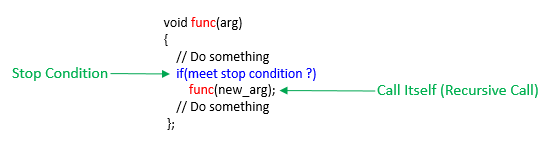Now let's look into a very simple (probably the simplest) recursive call example. The function definition is as bebelow. As you see, the printNum(num) function calls itself in the body with the new parameter of 'num + 1'. One important thing to note here is that in recursive call, you normally passes the parameters with the changed value especially when the parameter will be used as a stopping condition check.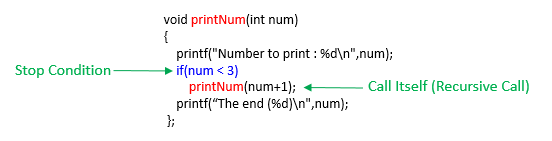Now assume that you call the function as printNum(1). What would happen ? This is what I wanted to show in Example 1. The each step of what will happen is described as below. I am pretty sure that it may not clear to you with just single read and you may get even more confused. But don't worry..  just follow through the simple examples in this page line by line and then create your own program with using recursive function. You will get familiar with this tricks gradually.. but it will take long time. You may say.. if the recursive function is doing the same thing over and over, why not using for() or while() loop. Definately, in many recursive functions can be replaced by for() or while() loop, but you may see in the future some cases where it is very difficult or really messy to implement if you use for() or while() loop instead of recursive function.  For now, you just assume that you definitely need to write a recursive function (e.g, for school homework :)) and let's go through our simple example.   Step 1 : Following is the status where the first function call PrintNum(1) occurred.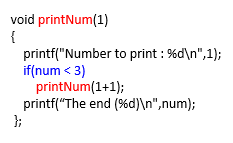Step 2 : Since the stop condition is not met, it call the function itself (do recursive call) with changed argment, printNum(1+1). As a result, new memory location is reserved for the new call as shown below.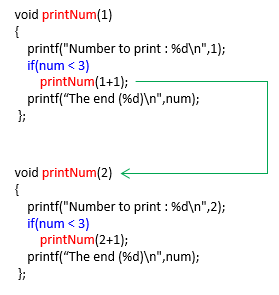Step 3 : Since the stop condition is not met, it call the function itself (do recursive call) with changed argment, printNum(2+1). As a result, new memory location is reserved for the new call as shown below.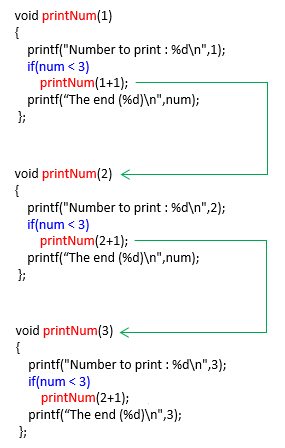Step 4 : Now the argument value at step 3 meets stop condition and does not do recursive call and proceed to the end of the function as shown below.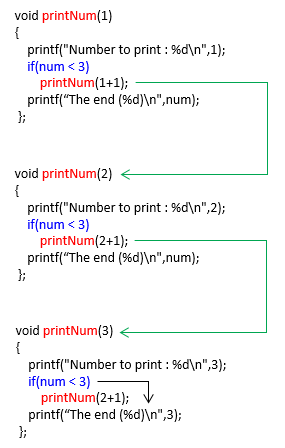Step 5 :  Since it reached the end of one of the function, it retruns and goes back to the previous function and perform the remaining procedure as illustrated below.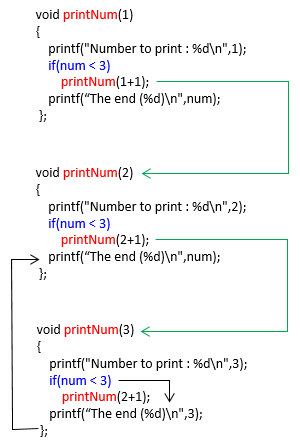Step 6 :  Now the execution of the last called function is complete and removed from the memory. And the second last function reached the end and return and get back to the remaining part of the previous call as illustrated below.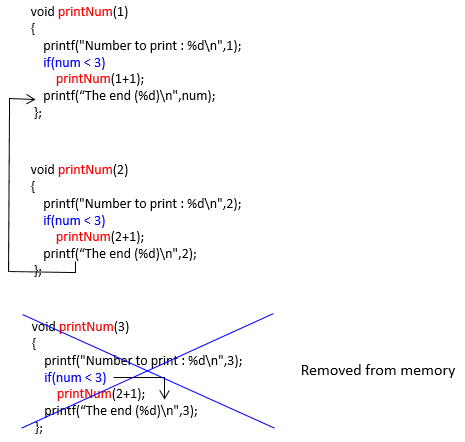Step 7 :  Now the execution of the second last called function is complete and removed from the memory. The previous function (the very first function call in this example) reachech the end and return. This completes the whole recursive calls.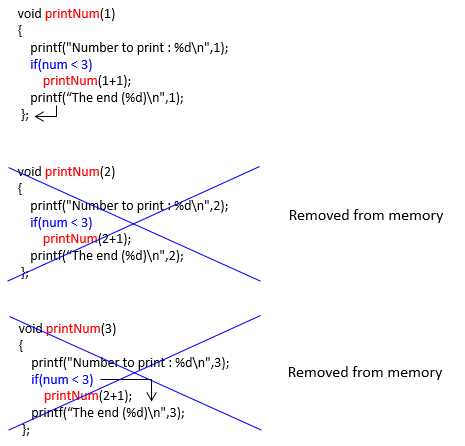Example 1 > ----------------------------------------------------------------------------------------------   #include   void printNum(int num) {     printf("Number to print : %d\n",num);     if(num < 10)         printNum(num+1);          };   int main(int argc, char* argv[]) {       printNum(1);       return 0; }   Result > Number to print : 1 Number to print : 2 Number to print : 3 Number to print : 4 Number to print : 5 Number to print : 6 Number to print : 7 Number to print : 8 Number to print : 9 Number to print : 10   Example 2 > ----------------------------------------------------------------------------------------------   #include   void printNum(int num) {     printf("Number to print : %d\n",num);     if(num < 10) {         printf("-->Calling printNum(%d)\n",num+1);         printNum(num+1);     };     printf("==>Reaching the end of function(%d)\n",num);         };   int main(int argc, char* argv[]) {       printNum(1);     return 0; }   Result > Number to print : 1 -->Calling printNum(2) Number to print : 2 -->Calling printNum(3) Number to print : 3 -->Calling printNum(4) Number to print : 4 -->Calling printNum(5) Number to print : 5 -->Calling printNum(6) Number to print : 6 -->Calling printNum(7) Number to print : 7 -->Calling printNum(8) Number to print : 8 -->Calling printNum(9) Number to print : 9 -->Calling printNum(10) Number to print : 10 ==>Reaching the end of function(10) ==>Reaching the end of function(9) ==>Reaching the end of function(8) ==>Reaching the end of function(7) ==>Reaching the end of function(6) ==>Reaching the end of function(5) ==>Reaching the end of function(4) ==>Reaching the end of function(3) ==>Reaching the end of function(2) ==>Reaching the end of function(1)     Example 3 > ----------------------------------------------------------------------------------------------   #include   void printNum(int num, int maxNum) {     printf("Number to print : %d\n",num);     if(num < maxNum) {         printf("-->Calling printNum(%d,%d)\n",num+1,maxNum);         printNum(num+1,maxNum);     };     printf("==>Reaching the end of function(%d)\n",num);         };   int main(int argc, char* argv[]) {       printNum(1,10);     return 0; }   Result > Number to print : 1 -->Calling printNum(2,10) Number to print : 2 -->Calling printNum(3,10) Number to print : 3 -->Calling printNum(4,10) Number to print : 4 -->Calling printNum(5,10) Number to print : 5 -->Calling printNum(6,10) Number to print : 6 -->Calling printNum(7,10) Number to print : 7 -->Calling printNum(8,10) Number to print : 8 -->Calling printNum(9,10) Number to print : 9 -->Calling printNum(10,10) Number to print : 10 ==>Reaching the end of function(10) ==>Reaching the end of function(9) ==>Reaching the end of function(8) ==>Reaching the end of function(7) ==>Reaching the end of function(6) ==>Reaching the end of function(5) ==>Reaching the end of function(4) ==>Reaching the end of function(3) ==>Reaching the end of function(2) ==>Reaching the end of function(1)